## Monday, December 31, 2018

2013
(May)
MATHEMATICS
(Major)
Course: 401
[A: Computer Programming (C Programming)]
Full Marks: 50
Pass Marks: 20
Time: 2 ½ hours
The figures in the margin indicate full marks for the questions

1. Choose the correct answer: 1x5=5
1. The maximum length of a character constant can be
1. 1 character.
2. 8 characters.
3. 254 characters.
4. None of the above.
1. If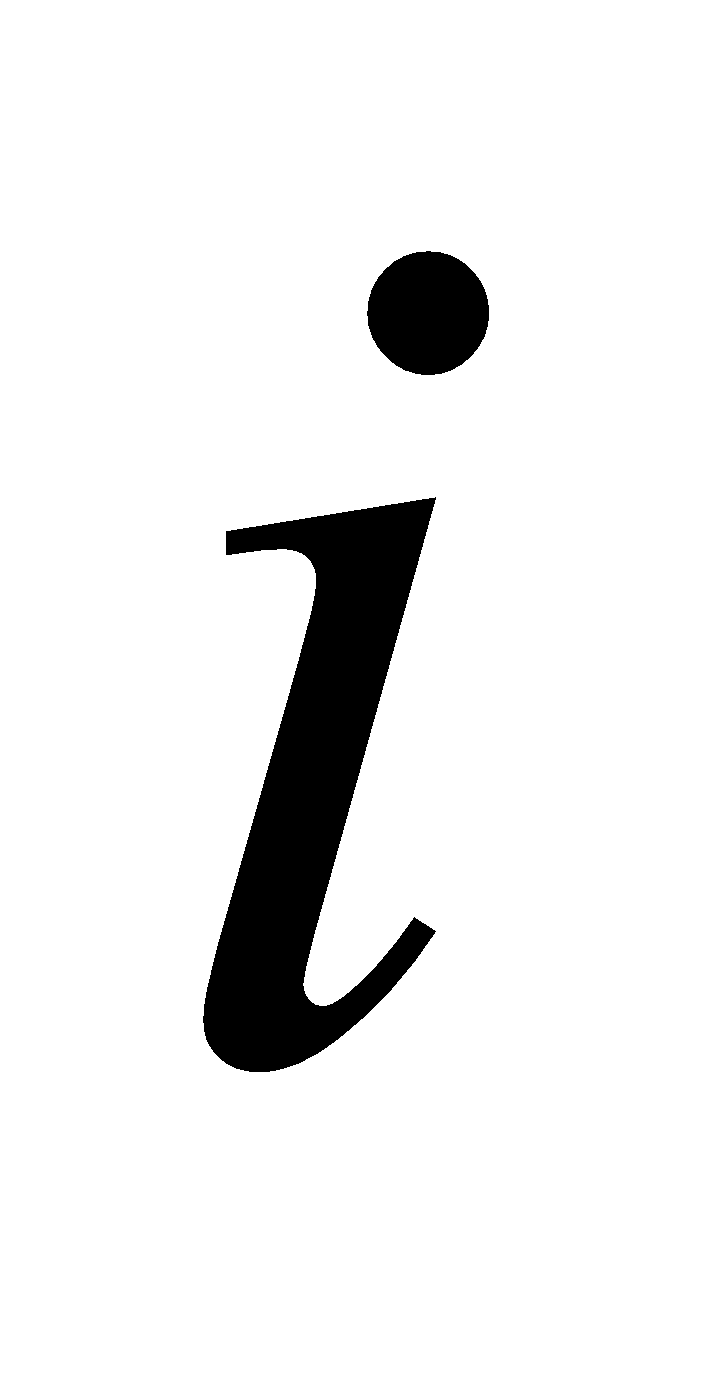is an integer variable,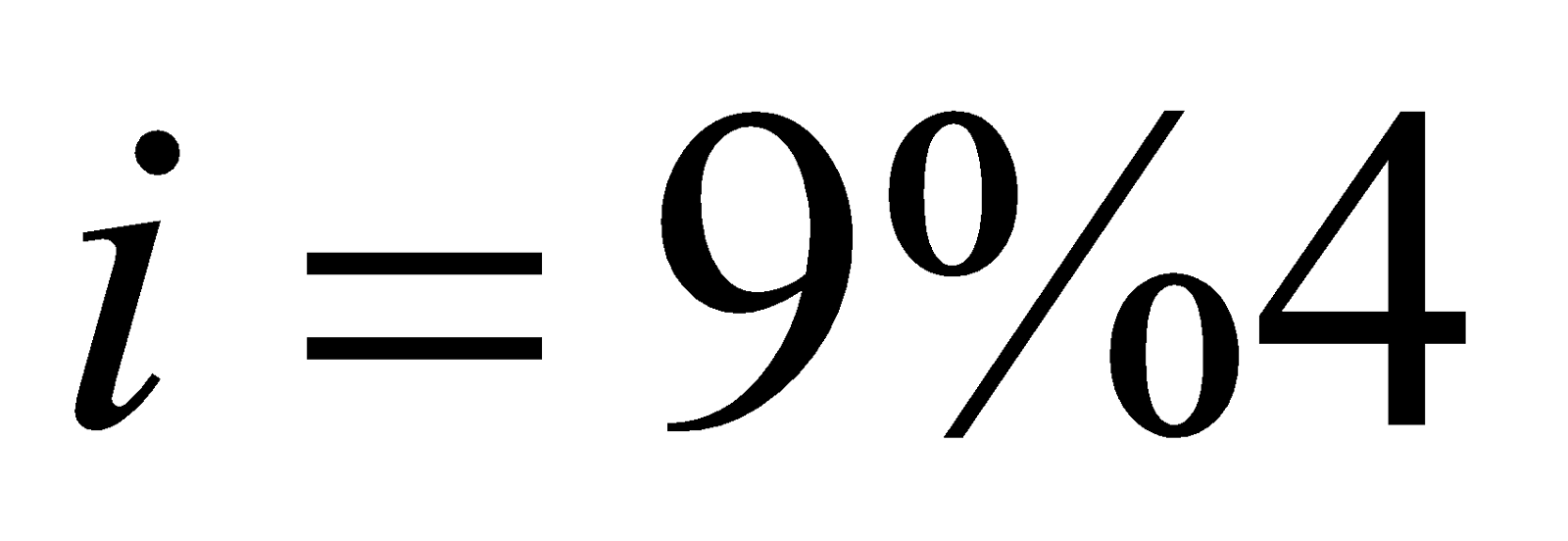will return a value
1. 2
2. 1
3. 2.25
4. 0
1. A DO/WHILE loop is useful only when we want that the statements within the loop must be executed
1. Only once.
2. More than once.
3. At least once.
4. None of the above.
1. The keyword used to transfer control from a function back to the calling function is
1. Switch.
2. Goto.
3. Goback.
4. Return.
1. An alphabet.
2. A number.
3. A special symbol other than underscore.
4. Both (ii) and (iii) above.
2. Answer the following questions: 2x8=16
1. Name the basic data types in C.
2. Write a short note on algorithm.
3. Write the modes of expressions in C.
4. Mention the bitwise operators in C.
5. Mention the programming situations in which the logical operators && and 11 are useful.
6. Write the printf statement to print the following: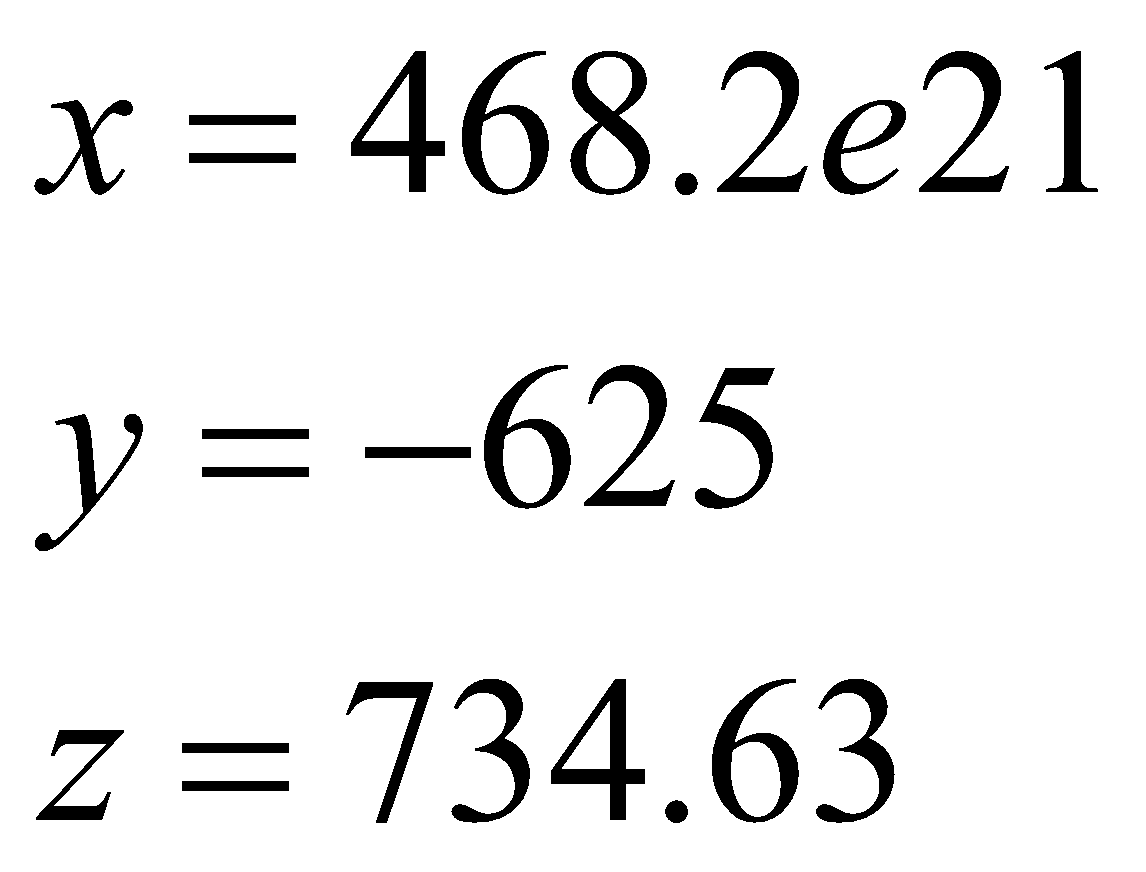1. Let two numbers be entered through the keyboard into two locations. Write a C program to interchange the contents of the variables.
2. Write the syntax of ‘if else’ statement and draw flowchart for it.
3. What is flowchart? Draw a flowchart to calculate the largest number among three given numbers. 1+3=4
4. Write a C program to calculate the sum of the digits of a four-digit integer number. 3
Or
Write a C program to find the solution of the system of linear equations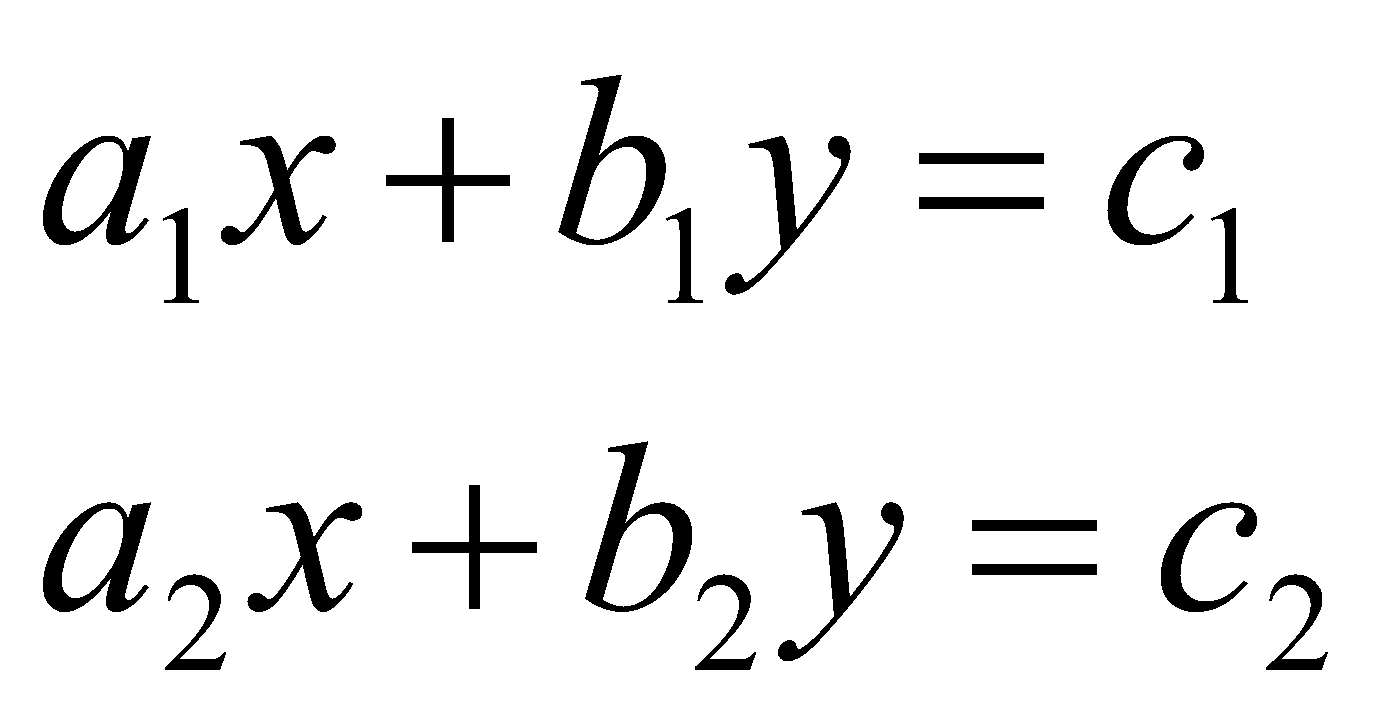5. Write a C program to calculate compound interest. 4
Or
Write a C program to check whether a triangle is valid or not, when the three angles of the triangle are entered through the keyboard. (A triangle is valid if the sum of the three angles is equal to 180 degrees.)
6. Define an array. Write a program to find average marks of 100 students in a test. 1+3=4
Or
Write a C program to find the mean and standard deviation of a one-dimensional array of n numbers. 4
7. Write a program to generate first 20 Fibonacci numbers   1, 1, 2, 3, 5, 8,….. 3
Or
Write a C program to arrange an array of 10 numbers in ascending order.
8. Write a C program to multiply two 3 x 3 matrices. 4
Or
Write a C program to determine whether a given number is prime or not.
9. What is function? Write a C program to find square of a number using function prototype. 1+2=3
10. Write a C program to evaluate factorial of a number using function prototype. 4
Or
Write a C program to find the solution of the quadratic equation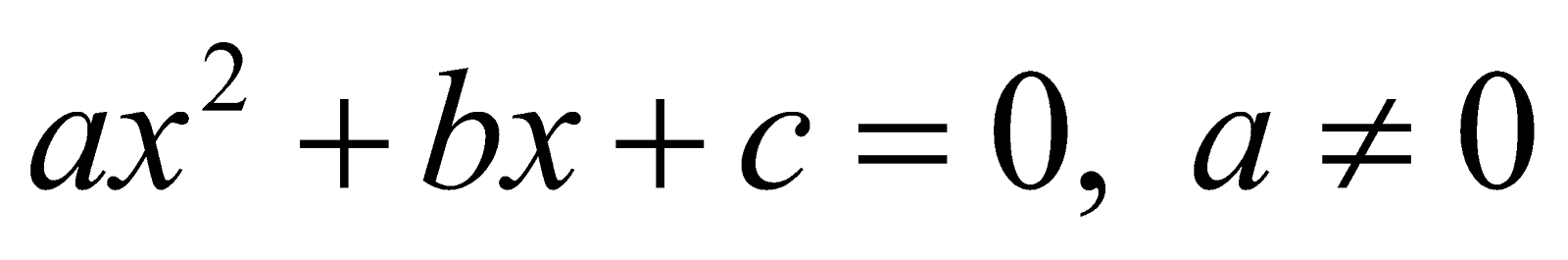***